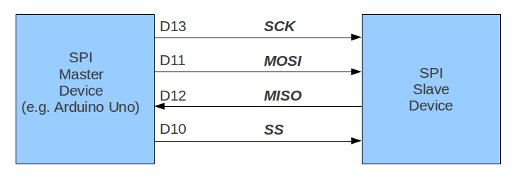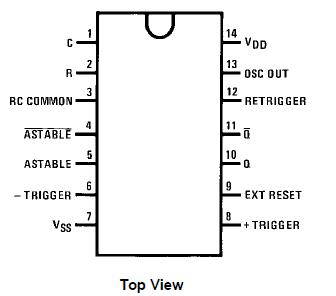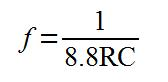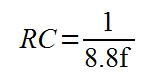# Author Archives: Cindy WuStaff writer and technical support for PMD Way Limited (pmdway.com) and tronixstuff.com

# Tutorial: Arduino and the SPI bus

This is the first of two chapters in which we are going to start investigating the SPI data bus, and how we can control devices using it with our Arduino systems.

The SPI bus may seem to be a complex interface to master, however with some brief study of this explanation and practical examples you will soon become a bus master! To do this we will learn the necessary theory, and then apply it by controlling a variety of devices. In this tutorial things will be kept as simple as possible.

But first of all, what is it? And some theory…

SPI is an acronym for “Serial Peripheral Interface”. It is a synchronous serial data bus – data can travel in both directions at the same time, as opposed to (for example) the I2C bus that cannot do so. To allow synchronous data transmission, the SPI bus uses four wires. They are called:

• MOSI – Master-out, Slave-in. This line carries data from our Arduino to the SPI-controlled device(s);
• MISO – Master-in, Slave out. This line carries data from the SPI-controlled device(s) back to the Arduino;
• SS – Slave-select. This line tells the device on the bus we wish to communicate with it. Each SPI device needs a unique SS line back to the Arduino;
• SCK – Serial clock.

Within these tutorials we consider the Arduino board to be the master and the SPI devices to be slaves. On our Arduino Uno and compatible boards the pins used are:

• SS – digital 10. You can use other digital pins, but 10 is generally the default as it is next to the other SPI pins;
• MOSI – digital 11;
• MISO – digital 12;
• SCK – digital 13;

Arduino Mega users – MISO is 50, MOSI is 51, SCK is 52 and SS is usually 53. If you are using an Arduino Leonardo, the SPI pins are on the ICSP header pins. See here for more information. You can control one or more devices with the SPI bus. For example, for one device the wiring would be:Data travels back and forth along the MOSI and MISO lines between our Arduino and the SPI device. This can only happen when the SS line is set to LOW. In other words, to communicate with a particular SPI device on the bus, we set the SS line to that device to LOW, then communicate with it, then set the line back to HIGH. If we have two or more SPI devices on the bus, the wiring would resemble the following:Notice how there are two SS lines – we need one for each SPI device on the bus. You can use any free digital output pin on your Arduino as an SS line. Just remember to have all SS lines high except for the line connected to the SPI device you wish to use at the time.

Data is sent to the SPI device in byte form. You should know by now that eight bits make one byte, therefore representing a binary number with a value of between zero and 255.

When communicating with our SPI devices, we need to know which way the device deals with the data – MSB or LSB first. MSB (most significant bit) is the left-hand side of the binary number, and LSB (least significant bit) is the right-hand side of the number. That is:Apart from sending numerical values along the SPI bus, binary numbers can also represent commands. You can represent eight on/off settings using one byte of data, so a device’s parameters can be set by sending a byte of data. These parameters will vary with each device and should be illustrated in the particular device’s data sheet. For example, a digital potentiometer IC with six pots:This device requires two bytes of data. The ADDR byte tells the device which of six potentiometers to control (numbered 0 to 5), and the DATA byte is the value for the potentiometer (0~255). We can use integers to represent these two values. For example, to set potentiometer number two to 125, we would send 2 then 125 to the device.

How do we send data to SPI devices in our sketches?

First of all, we need to use the SPI library. It is included with the default Arduino IDE installation, so put the following at the start of your sketch:

`#include "SPI.h"`

Next, in void.setup() declare which pin(s) will be used for SS and set them as OUTPUT. For example,

`pinMode(ss, OUTPUT);`

where ss has previously been declared as an integer of value ten. Now, to activate the SPI bus:

`SPI.begin();`

and finally we need to tell the sketch which way to send data, MSB or LSB first by using

`SPI.setBitOrder(MSBFIRST);`

or

`SPI.setBitOrder(LSBFIRST);`

When it is time to send data down the SPI bus to our device, three things need to happen. First, set the digital pin with SS to low:

`digitalWrite(SS, LOW);`

Then send the data in bytes, one byte at a time using:

`SPI.transfer(value);`

Value can be an integer/byte between zero and 255. Finally, when finished sending data to your device, end the transmission by setting SS high:

`digitalWrite(ss, HIGH);`

Sending data is quite simple. Generally the most difficult part for people is interpreting the device data sheet to understand how commands and data need to be structured for transmission. But with some practice, these small hurdles can be overcome.

Now for some practical examples!

Time to get on the SPI bus and control some devices. By following the examples below, you should gain a practical understanding of how the SPI bus and devices can be used with our Arduino boards.

MCP4162 Example

Our first example will use a simple yet interesting part – a digital potentiometer (we also used one in the I2C tutorial). This time we have a Microchip MCP4162-series 10k rheostat:Here is the data sheet for your perusal. To control it we need to send two bytes of data – the first byte is the control byte, and thankfully for this example it is always zero (as the address for the wiper value is 00h [see table 4-1 of the data sheet]).  The second byte is the the value to set the wiper, which controls the resistance. So to set the wiper we need to do three things in our sketch…

First, set the SS (slave select) line to low:

`digitalWrite(10, LOW);`

Then send the two byes of data:

```SPI.transfer(0); // command byte
SPI.transfer(value); // wiper value```

Finally set the SS line back to high:

`digitalWrite(10, HIGH);`

Easily done. Connection to our Arduino board is very simple – consider the MCP4162 pinout:Vdd connects to 5V, Vss to GND, CS to digital 10, SCK to digital 13, SDI to digital 11 and SDO to digital 12. Now let’s run through the available values of the MCP4162 in the following sketch:

```/*
SPI bus demo using a Microchip MCP4162 digital potentiometer [http://bit.ly/iwDmnd]
*/

#include "SPI.h" // necessary library
int ss=10; // using digital pin 10 for SPI slave select
int del=200; // used for various delays

void setup()
{
pinMode(ss, OUTPUT); // we use this for SS pin
SPI.begin(); // wake up the SPI bus.
SPI.setBitOrder(MSBFIRST);
// our MCP4162 requires data to be sent MSB (most significant byte) first
}

void setValue(int value)
{
digitalWrite(ss, LOW);
SPI.transfer(0); // send command byte
SPI.transfer(value); // send value (0~255)
digitalWrite(ss, HIGH);
}

void loop()
{
for (int a=0; a<256; a++)
{
setValue(a);
delay(del);
}
for (int a=255; a>=0; --a)
{
setValue(a);
delay(del);
}
}```

Now to see the results of the sketch. In the following video clip, a we run up through the resistance range and measure the rheostat value with a multimeter:

Before moving forward, if digital potentiometers are new for you, consider reading this short guide written by Microchip about the differences between mechanical and digital potentiometers.

Another example:

In this example, we will use the Analog Devices AD5204 four-channel digital potentiometer (data sheet.pdf). It contains four 10k ohm linear potentiometers, and each potentiometer is adjustable to one of 256 positions.

The settings are volatile, which means they are not remembered when the power is turned off. Therefore when power is applied the potentiometers are all pre set to the middle of the scale. Our example is the SOIC-24 surface mount example, however it is also manufactured in DIP format as well.To make life easier it can be soldered onto a SOIC breakout board which converts it to a through-hole package:In this example, we will control the brightness of four LEDs. Wiring is very simple. Pinouts are in the data sheet.And the sketch:

```#include <SPI.h> // necessary library
int ss=10; // using digital pin 10 for SPI slave select
int del=5; // used for fading delay
void setup()
{
pinMode(ss, OUTPUT); // we use this for SS pin
SPI.begin(); // wake up the SPI bus.
SPI.setBitOrder(MSBFIRST);
// our AD5204 requires data to be sent MSB (most significant byte) first. See data sheet page 5
allOff(); // we do this as pot memories are volatile
}
void allOff()
// sets all potentiometers to minimum value
{
for (int z=0; z<4; z++)
{
setPot(z,0);
}
}
void allOn()
// sets all potentiometers to maximum value
{
for (int z=0; z<4; z++)
{
setPot(z,255);
}
}
void setPot(int pot, int level)
// sets potentiometer 'pot' to level 'level'
{
digitalWrite(ss, LOW);
SPI.transfer(pot);
SPI.transfer(level);
digitalWrite(ss, HIGH);
}
{
for (int z=0; z
{
for (int a=0; a<4; a++)
{
for (int l=0; l<255; l++)
{
setPot(a,l);
delay(del);
}
for (int l=255; l>=0; --l)
{
setPot(a,l);
delay(del);
}
}
}
{
for (int a=0; a<count; a++)="" {="" for="" (int="" l="0;" l<255;="" l++)="" setpot(0,l);="" setpot(1,l);="" setpot(2,l);="" setpot(3,l);="" delay(del);="" }="">=0; --l)
{
setPot(0,l);
setPot(1,l);
setPot(2,l);
setPot(3,l);
delay(del);
}
}
}
void loop()
{
delay(1000);
}```

The function allOff() and allOn() are used to set the potentiometers to minimum and maximum respectively. We use allOff() at the start of the sketch to turn the LEDs off. This is necessary as on power-up the wipers are generally set half-way.

Furthermore we use them in the blinkAll() function to … blink the LEDs. The function setPot() accepts a wiper number (0~3) and value to set that wiper (0~255). Finally the function indFade() does a nice job of fading each LED on and off in order – causing an effect very similar to pulse-width modulation.

Finally, here it is in action:

So there you have it – hopefully an easy to understand introduction to the world of the SPI bus and how to control the devices within. As always, now it is up to you and your imagination to find something to control or get up to other shenanigans. In the next SPI article we will look at reading and writing data via the SPI bus.

This post brought to you by pmdway.com – everything for makers and electronics enthusiasts, with free delivery worldwide.

To keep up to date with new posts at tronixstuff.com, please subscribe to the mailing list in the box on the right, or follow us on twitter @tronixstuff.

# Tutorial: Your Arduino’s inbuilt EEPROM

In this article we are going to examine the internal EEPROM in our Arduino boards. What is an EEPROM some of you may be saying? An EEPROM is an Electrically Erasable Programmable Read-Only Memory.

It is a form of non-volatile memory that can remember things with the power being turned off, or after resetting the Arduino. The beauty of this kind of memory is that we can store data generated within a sketch on a more permanent basis.

Why would you use the internal EEPROM?

For situations where data that is unique to a situation needs a more permanent home. For example, storing the unique serial number and manufacturing date of a commercial Arduino-based project – a function of the sketch could display the serial number on an LCD, or the data could be read by uploading a ‘service sketch’. Or you may need to count certain events and not allow the user to reset them – such as an odometer or operation cycle-counter.

What sort of data can be stored?

Anything that can be represented as bytes of data. One byte of data is made up of eight bits of data. A bit can be either on (value 1) or off (value 0), and are perfect for representing numbers in binary form. In other words, a binary number can only uses zeros and ones to represent a value. Thus binary is also known as “base-2″, as it can only use two digits.

How can a binary number with only the use of two digits represent a larger number? It uses a lot of ones and zeros. Let’s examine a binary number, say 10101010. As this is a base-2 number, each digit represents 2 to the power of x, from x=0 onwards:See how each digit of the binary number can represent a base-10 number. So the binary number above represents 85 in base-10 – the value 85 is the sum of the base-10 values. Another example – 11111111 in binary equals 255 in base 10.Now each digit in that binary number uses one ‘bit’ of memory, and eight bits make a byte. Due to internal limitations of the microcontrollers in our Arduino boards, we can only store 8-bit numbers (one byte) in the EEPROM.

This limits the decimal value of the number to fall between zero and 255. It is then up to you to decide how your data can be represented with that number range. Don’t let that put you off – numbers arranged in the correct way can represent almost anything!

There is one limitation to take heed of – the number of times we can read or write to the EEPROM. According to the manufacturer Atmel, the EEPROM is good for 100,000 read/write cycles (see the data sheet).

One would suspect this to be a conservative estimate, however you should plan accordingly. *Update* After some experimentation, the life proved to be a lot longer

Now we know our bits and and bytes, how many bytes can be store in our Arduino’s microcontroller? The answer varies depending on the model of microcontroller. For example:

• Boards with an Atmel ATmega328, such as Arduino Uno, Uno SMD, Nano, Lilypad, etc. – 1024 bytes (1 kilobyte)
• Boards with an Atmel ATmega1280 or 2560, such as the Arduino Mega series – 4096 bytes (4 kilobytes)
• Boards with an Atmel ATmega168, such as the original Arduino Lilypad, old Nano, Diecimila etc – 512 bytes.

If you are unsure have a look at the Arduino hardware index or ask your board supplier.

If you need more EEPROM storage than what is available with your microcontroller, consider using an external I2C EEPROM as described in the Arduino and I2C tutorial part two.

At this point we now understand what sort of data and how much can be stored in our Arduino’s EEPROM. Now it is time to put this into action. As discussed earlier, there is a finite amount of space for our data. In the following examples, we will use a typical Arduino board with the ATmega328 with 1024 bytes of EEPROM storage.

To use the EEPROM, a library is required, so use the following library in your sketches:

`#include "EEPROM.h"`

The rest is very simple. To store a piece of data, we use the following function:

`EEPROM.write(a,b);`

The parameter a is the position in the EEPROM to store the integer (0~255) of data b. In this example, we have 1024 bytes of memory storage, so the value of a is between 0 and 1023. To retrieve a piece of data is equally as simple, use:

`z = EEPROM.read(a);`

Where z is an integer to store the data from the EEPROM position a. Now to see an example.

This sketch will create random numbers between 0 and 255, store them in the EEPROM, then retrieve and display them on the serial monitor. The variable EEsize is the upper limit of your EEPROM size, so (for example) this would be 1024 for an Arduino Uno, or 4096 for a Mega.

```// Arduino internal EEPROM demonstration

#include <EEPROM.h>
int zz;
int EEsize = 1024; // size in bytes of your board's EEPROM

void setup()
{
Serial.begin(9600);
}
void loop()
{
Serial.println("Writing random numbers...");
for (int i = 0; i < EEsize; i++)
{
zz=random(255);
EEPROM.write(i, zz);
}
Serial.println();
for (int a=0; a<EEsize; a++)
{
Serial.print("EEPROM position: ");
Serial.print(a);
Serial.print(" contains ");
Serial.println(zz);
delay(25);
}
}```

The output from the serial monitor will appear as such:So there you have it, another useful way to store data with our Arduino systems. Although not the most exciting tutorial, it is certainly a useful.

This post brought to you by pmdway.com – everything for makers and electronics enthusiasts, with free delivery worldwide.

To keep up to date with new posts at tronixstuff.com, please subscribe to the mailing list in the box on the right, or follow us on twitter @tronixstuff.

# Review – CD4047 Astable/Monostable Multivibrator

In this article we are going to examine an older but still highly useful integrated circuit – the 4047 Astable/Monostable multivibrator:Our reason for doing this is to demonstrate another way to create a square-wave output for digital circuits (astable mode) and also generate single pulses (monostable mode).

Sometimes one can get carried away with using a microcontroller by default – and forget that there often can be simpler and much cheaper ways of doing things. And finally, the two can often work together to solve a problem.

What is a multivibrator? In electronics terms this means more than one vibrator. It creates an electrical signal that changes state on a regular basis (astable) or on demand (monostable).

You may recall creating monostable and astable timers using the 555 timer described in an earlier article. One of the benefits of the 4047 is being able to do so as well, but with fewer external components. Here is the pinout diagram for a 4047 (from the Fairchild data sheet):Note that there are three outputs, Q, Q and OSC out. Q is the normal output, Q is the inverse of Q – that is if Q is high, Q is low – at the same frequency. OSC output provides a signal that is very close to twice the frequency of Q. We will consider the other pins as we go along. In the following small video, we have LEDs connected to all three outputs – you can see how Q and Q alternate, and the increased frequency of OSC out:

That was an example of the astable mode.  The circuit used is shown below. The only drawback of using a 4047 is that you cannot alter the duty cycle of your astable output – it will always be 50% high and 50% low. The oscillator output is not guaranteed to have a 50% duty cycle, but comes close. The time period (and therefore the frequency) is determined by two components – R1 and the capacitor:

[Quick update – in the schematic below, also connect 4047 pin 14 to +5V]The values for R2~R4 are 560 ohms, for the LEDs. R1 and the capacitor form an RC circuit, which controls the oscillation frequency. How can we calculate the frequency? The data sheet tells us that time (period of time the oscillator is ‘high’) is equal to 4.4 multiplied by the value of R1 and the capacitor. As the duty cycle is always 50%, we double this value, then divide the result into one. In other words:And as the frequency from the OSC out pin is twice that of Q or Q, the formula for the OSC out frequency is:However the most useful formula would allow you to work with the values of R and C to use for a desired frequency f:When calculating your values, remember that you need to work with whole units, such as Farads and Ohms- not microfarads, mega-ohms, etc. This chart of SI prefixes may be useful for conversions.

The only thing to take note of is the tolerance of your resistor and capacitor. If you require a certain, exact frequency try to use some low-tolerance capacitors, or replace the resistor with a trimpot of a value just over your required resistor value.

Then you can make adjustments and measure the result with a frequency counter. For example, when using a value of 0.1uF for C and 15 k ohm for R, the theoretical frequency is 151.51 Hz; however in practice this resulted with a frequency of 144.78 Hz.

Don’t forget that the duty cycle is not guaranteed to be 50% from the OSC out pin. This is shown in the following demonstration video. We measure the frequency from all three output pins, then measure the duty cycle from the same pins:

(The auto-ranging on that multimeter is somewhat annoying).

Now for some more more explanation about the 4047. You can activate the oscillations in two ways, via a high signal into pin 5 (pin 4 must then be low) or via a low signal into pin 4 (and pin 5 must be low). Setting pin 9 high will reset the oscillator, so Q is low and Q is high.

The monostable mode is also simple to create and activate. I have not made a video clip of monstable operation, as this would only comprise of staring at an LED. However, here is an example circuit with two buttons added, one to trigger the pulse (or start it), and another to reset the timer (cancel any pulse and start again):

[Quick update – in the schematic below, also connect 4047 pin 14 to +5V]The following formula is used to calculate the duration of the pulse time:Where time is in seconds, R is Ohms, and C is Farads. Once again, the OSC output pin also has a modified output – it’s time period will be 1.2RC.

To conclude, the 4047 offers a simple and cheap way to generate a 50% duty cycle  square wave or use as a monostable timer. You can order your 4047s from PMD Way with free delivery worldwide.

This post brought to you by pmdway.com – everything for makers and electronics enthusiasts, with free delivery worldwide.

To keep up to date with new posts at tronixstuff.com, please subscribe to the mailing list in the box on the right, or follow us on twitter @tronixstuff.